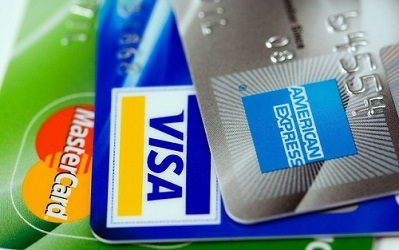Select Page
October 21, 2013

# How to calculate interest on credit card balance?One of the easiest way of shopping these days is by using our credit cards. Reason is, we buy now but pay later. But at the same time have you noticed or understood the way the interest is calculated on such transactions? We hardly take notice of the calculation method used.

Reason for such neglect is, we mainly concentrate on terms such as billing cycle, grace period we get, minimum balance amount or the annual charges. But forget to notice the rate of interest or the method of interest calculation. Hence, it is better to understand it. I try to simplify it with a simple example.In India lot of card providers usually use the method of calculation called Average Daily Balance Method. Here in this method of calculation each day’s transaction will matter most for calculating interest on how much you owe. Let us say Mr. Arun has an XYZ bank credit card. Billing cycle will be 30 days. Assume annual interest levying will be 24%. He started the billing cycle with the balance of Rs.25,000. On the 10th day of the billing cycle he purchased some household goods worth of Rs.8,000. On the 20th day of the billing cycle he paid an amount of Rs.10, 000 to your credit card billing cycle. Now calculation will be done in below steps.

1) Calculating the average balance for the 30 day period.

From 1st day to 9th day balance is Rs.10,000. So we need to add this balance for the 9 days like Rs.10,000+Rs.10,000+Rs.10,000+Rs.10,000+Rs.10,000+Rs.10,000+Rs.10,000+Rs.10,000+Rs.10,000=Rs.90,000.

From 10th day to 19th day of billing cycle the balance will be Rs.10,000+Rs.5,000=Rs.15,000. So total balance for the period will be Rs.15,000+Rs.15,000+Rs.15,000+Rs.15,000+Rs.15,000+Rs.15,000+Rs.15,000+Rs.15,000+Rs.15,000+Rs.15,000=Rs.1,50,000.

From 20th day to 30th day of billing cycle the balance will be Rs.Rs.15,000-Rs.7,000=Rs.8,000. So total balance for the period will be Rs.8,000+Rs.8,000+Rs.8,000+Rs.8,000+Rs.8,000+Rs.8,000+Rs.8,000+Rs.8,000+Rs.8,000+Rs.8,000+Rs.8,000=Rs.88,000.

Now the total average balance will be (Rs.90,000+Rs.1,50,000+Rs.88,000)/30 days=Rs.10,933.

2) Divide the number of days in a year into billing cycle days. In this case billing cycle is for the period of 30 days. Hence 30/365=0.0821.

3) Multiply this 0.0821 factor with annual interest chargeable on credit card i.e. 24% . So it is (0.0821)*(0.24)=0.197.

4) Final step will be multiplying this interest to his daily average balance, i.e. (0.197)*(Rs.10,933)=Rs.2,154. This will be the interest Mr.Arun need to pay.

The same step can be achieved by putting appropriate values in below formula.

Average daily balance method for credit card=(days in billing cycle/365) (Annual Percentage Rate) (Average Daily Balance).

But do remember that while calculating this average daily balance, few banks adds charges made during the month. If they add so then your average daily balance will increase. In return you need to pay higher interest. Hence make sure what type of calculation they are doing.